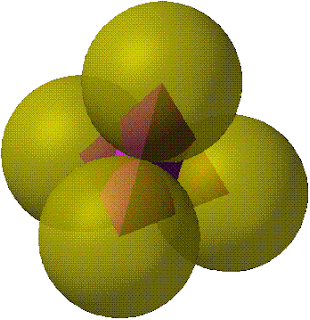## Monday, August 2, 2010

### Fox 54 - Discussion

Bob Ryden provided a solution below. This has been the first attempt to solve this fox. His solution has not been confirmed yet, but it is published here to start the discussion. Wanna say somethin', comment it out!
Let the radius of the spheres = 1.
Inside is a tetrahedron whose vertices are the centers
of the spheres. Its edge length = 2
and its volume = (1/8) √3On each face of the tetrahedron, build a triangular prism.
Volume of each prism = (1/2) 2 (√3) 1
Total volume of four prisms = 4√3On each edge of the tetrahedron, build a cylindrical sector.
The angle of the sector = 360 – 90 – 90 – dihedral angle of the tetrahedron
= 360 – 90 – 90 – arccos (1/3) = approx. 109.47°
Length of each cylindrical sector = 2, radius = 1
Total volume of the six sectors
= 6 π (1^2) 2 (109.47 / 360) ≈ 11.46Finally, there are pieces of the four original spheres that are not covered by any of the above. The four pieces together make one complete sphere, V = (4/3)π.

Total volume is the sum
tetrahedron + 4 prisms + 6 cylindrical sectors + sphere
= approx. 23.52